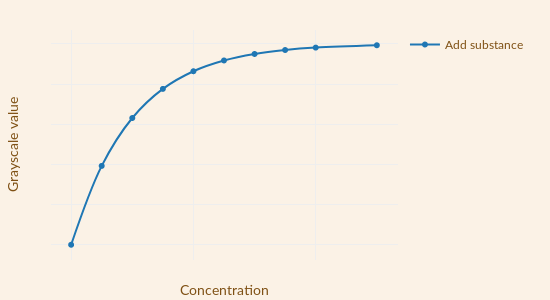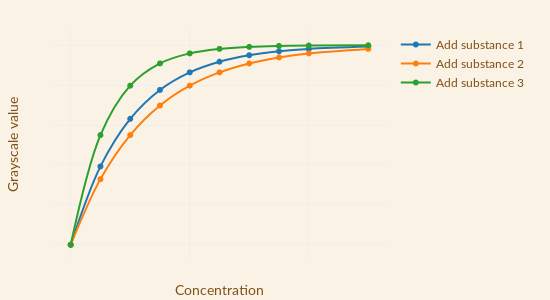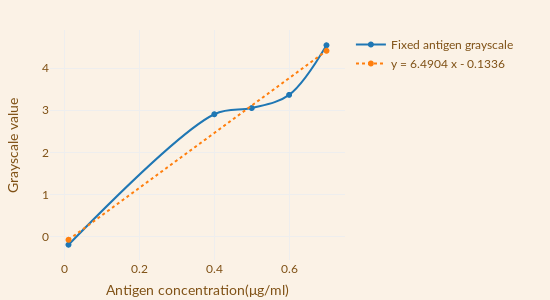# Team:DLUT China B/GrayValueConcentrationModel

Gray Value-Concentration Model

Gray Value-Concentration Model

By building a mathematical model, we demonstrate the principles of the project and provide guidance for optimizing product design and its application.

A grayscale model is built to solve the image quantization problem.

A kinetic model was established to solve the problem of time optimization of antigen fixation, reaction concentration ratio and detection time optimization.

The spatial perturbation model provides relevant conclusions about liquid crystal interference. A fixed antigen concentration threshold is given.

## The relationship between the gray value and the added substance concentration

To describe our results in antigen fixation, antibody ligation, and detection, a grayscale model was established. The model can more accurately reflect the results of the three phases. Especially in the test, the results can be digitized to provide better detection limits.

For the result of the gray value calculation, it is firstly grayscaled. Since it is a color picture, it needs to be grayed out first with Matlab. At the same time, in order to give a clear grayscale image, image equalization is performed and a balanced image is given[Attachment 1]. After obtaining the grayscale image, it is necessary to calculate the grayscale of the image using the formula (1). To make it easier to get accurate data, design a small software[Attachment 2] in a programming language that calculates the average grayscale of the image. There is an error in the data of the grayscale image. According to the experimental results, when the gray value is not too low, it is about 5%. The error is about 10% compared to the balanced grayscale image.

$$g_w = \sum_{i=0}^{255} i \times T_i \div T \tag{1}$$

For the experimental results, no matter which process. In the initial stage, the gray value is positively correlated with the concentration of the additive; as the additive concentration increases, the gray value is not proportional to the increase. There are two models that satisfy these properties, equation (2) and equation (3):

Michaelis-Menten model: $$y = \frac{\alpha x}{\beta + x} \tag{2}$$

Exponential growth model: $$y = \alpha (1- exp(-\beta x)) \tag{3}$$

Substituting the above two formulas into the relevant literature , where model (2) is very consistent. Simultaneous model (2) If the enzyme is compared to a slide, the substrate is similar to the additive. The resulting enzymatic reaction rate - substrate concentration and gray value - has a good fit to the added concentration. So using this model is very suitable. In other literature , the results obtained are also consistent. The relationship between gray value and concentration in this model is shown in Figure 1(a), which applies to multiple stages of the project. The different substances added are shown in Figure 2(b).(a) Addition of added substances(b) Relationship between different additions and gray values (joined separately)(c) Fixed antigen grayscale model curve Fig.1 Gray value and additive concentration relationship

In the step of immobilizing the antigen, other conditions are controlled and a large amount of experimental data is used to reduce the error. Experimental Results After data processing, Figure 1 (c), Equation $$\mathrm{Y_1}$$, can be obtained. The resulting image was used to predict other data and found good consistency. The established gray value - concentration model is consistent with the experiment. Therefore, the antibody immobilization model was further established and the result of the equation $$\mathrm{Z_1}$$ was obtained. It can be seen from the model and experimental data that the gray value is positively correlated with the additive concentration when the concentration is not large. It can be seen from the model and experimental data that the gray value is positively correlated with the additive concentration when the concentration is not large and is greater than 0.4 μg/ml.

$$y = \frac{\alpha}{\beta} x \quad \mathrm{(Ψ_q)}$$

$$y = 6.4904 x \quad \mathrm{(Y_1)}$$

$$y = 9.3506 x \quad \mathrm{(Z_1)}$$

When the concentration of the additive is not very large, the formula (2) can be changed to the formula $$\mathrm{Ψ_q}$$.

Grayscale values are also available when the slide is fixed to anything. Therefore, it is meaningful to perform statistics on the gray value of the unfixed antigen. The $$\mathrm{Y_1}$$ equation is the result of correcting the zero point deviation and comparing the relationship $$\mathrm{Ψ_q}$$. $$\mathrm{Z_1}$$ can be obtained by treating the modified antibody model in the same manner.

Zhang Jiao, Su Xiuxia, Yang Dong, Luan Chonglin,Label-free liquid crystal biosensor for cecropin B detection,Talanta,Volume 186,2018,Pages 60-64,ISSN 0039-9140, https://doi.org/10.1016/j.talanta.2018.04.004.

Li Xian, Li Guang, Yang Meng, Chen Long-Cong, Xiong Xing-Liang,Gold nanoparticle based signal enhancement liquid crystal biosensors for tyrosine assays,Sensors and Actuators B: Chemical,Volume 215,2015,Pages 152-158,ISSN 0925-4005, https://doi.org/10.1016/j.snb.2015.03.054.

[Attachment 1] Name:(Picture grayscale processing code) https://github.com/zkingri/gray

[Attachment 2] Except for all documents in Annex 1. https://github.com/zkingri/gray